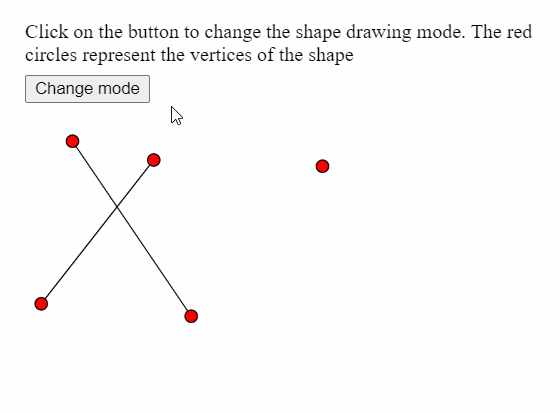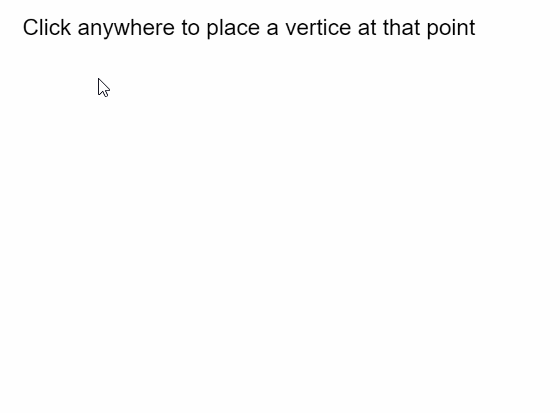Open in App
Not now

# p5.js | vertex() Function

• Last Updated : 31 May, 2020

The vertex() function in p5.js is used to specify the coordinates of the vertices used to draw a shape. It can only be used with the beginShape() and endShape() functions to make various shapes and curves like points, lines, triangles, quads and polygons.

Syntax:

`vertex( x, y )`

OR

`vertex( x, y, z, [u], [v] )`

Parameters: This function accepts five parameters as mentioned above and described below:

• x: It is a number that specifies the x-coordinate of the vertex.
• y: It is a number that specifies the y-coordinate of the vertex.
• z: It is a number that specifies the z-coordinate of the vertex.
• u: It is a number that specifies the u-coordinate of the texture of the vertex. It is an optional parameter.
• v: It is a number that specifies the v-coordinate of the texture of the vertex. It is an optional parameter.

The examples below illustrates the vertex() function in p5.js:

Example 1:

 `let currMode; ` ` `  `function` `setup() { ` `  ``createCanvas(400, 300); ` `  ``textSize(18); ` ` `  `  ``let shapeModes = [LINES, TRIANGLES, TRIANGLE_FAN,  ` `                    ``TRIANGLE_STRIP, QUADS]; ` `  ``let index = 0; ` `  ``currMode = shapeModes[index]; ` ` `  `  ``let helpText = createP( ` `    ```Click on the button to change the shape drawing mode. ` `    ``The red circles represent the vertices of the shape` ` `  ``); ` `  ``helpText.position(20, 0); ` ` `  `  ``let closeBtn = createButton(``"Change mode"``); ` `  ``closeBtn.position(20, 60); ` `  ``closeBtn.mouseClicked(() => { ` `    ``if` `(index < shapeModes.length) index++; ` `    ``else` `index = 0; ` `    ``currMode = shapeModes[index]; ` `  ``}); ` `} ` ` `  `function` `draw() { ` `  ``clear(); ` ` `  `  ``// Starting the shape using beginShape() ` `  ``beginShape(currMode); ` ` `  `  ``// Specifying all the vertices ` `  ``vertex(145, 245); ` `  ``vertex(50, 105); ` `  ``vertex(25, 235); ` `  ``vertex(115, 120); ` `  ``vertex(250, 125); ` ` `  `  ``// Ending the shape using endShape() ` `  ``endShape(); ` ` `  `  ``// Points for demonstration ` `  ``fill(``"red"``); ` `  ``circle(145, 245, 10); ` `  ``circle(50, 105, 10); ` `  ``circle(25, 235, 10); ` `  ``circle(115, 120, 10); ` `  ``circle(250, 125, 10); ` `  ``noFill(); ` `} `

Output:Example 2:

 `let vertices = []; ` ` `  `function` `setup() { ` `  ``createCanvas(400, 300); ` `  ``textSize(18); ` `  ``text(``"Click anywhere to place a vertice "``+ ` `       ``"at that point"``, 10, 20); ` `} ` ` `  `function` `mouseClicked() { ` `  ``// Update the vertices array with ` `  ``// current mouse position ` `  ``vertices.push({ x: mouseX, y: mouseY }); ` ` `  `  ``clear(); ` `  ``fill(``"black"``); ` `  ``text(``"Click anywhere to place a vertice "``+ ` `       ``"at that point"``, 10, 20); ` ` `  `  ``noFill(); ` ` `  `  ``// Draw shape using the current vertices array ` `  ``beginShape(); ` `  ``for` `(let i = 0; i < vertices.length; i++) ` `    ``vertex(vertices[i].x, vertices[i].y); ` `  ``endShape(CLOSE); ` ` `  `  ``fill(``"red"``); ` `  ``// Draw a circle at all the vertices ` `  ``for` `(let i = 0; i < vertices.length; i++) ` `    ``circle(vertices[i].x, vertices[i].y, 15); ` `} `

Output:Online editor: https://editor.p5js.org/

Reference: https://p5js.org/reference/#/p5/vertex

My Personal Notes arrow_drop_up
Related Articles